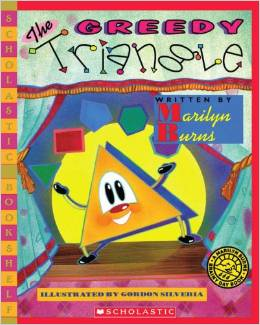# Describe 2D Shapes

6 teachers like this lesson
Print Lesson

## Objective

SWBAT describe essential attributes of two-dimensional shapes.

#### Big Idea

What’s the point? In this lesson students learn to describe the vertices and sides of a 2D shapes.

## Activating Strategy

10 minutes

I begin this lesson by reading the book “The Greedy Triangle”.  I like to use this book because it demonstrates the transformation of the triangle to other polygons based on the addition of essential attributes (sides and vertices).  While reading the book, I have students come up and count the vertices of the shapes.If the book isn't available, there is a YouTube version.

In this video, a student is counting the sides and vertices on a square.

## Teaching Strategies

15 minutes

I then display a trapezoid and a hexagon. Have children share their observations about each shape. Then ask questions to generate discussion about ways these shapes are alike or different from other shapes. For example:

• How is the trapezoid like a square? How is it different? (Both are two-dimensional shapes that have 4 sides and 4 vertices. All four sides of a trapezoid are not the same length.)

Exploring the attributes of shapes is a first step in developing geometrical ideas. As I work through this lesson, I help children relate what they are learning to the world around them. I like to ask where they have seen squares, rectangles, triangles, and circles outside of school, and how those shapes are like the shapes in their math lessons.  Geometry builds on children’s spatial sense, and many children who struggle with arithmetic may find the mathematics of geometry much more satisfying and interesting! I like to take advantage of this and get students excited and involved who otherwise tend to disengage during math.

One of the common student misconceptions in describing the attributes of geometric shapes (1.G.A.1) that I have found is that students may identify only the vertices on the trapezoid that have acute angles. Remind children that a vertex feels like a point, but it is still a vertex even if it is less pointy. A vertex is the point where two sides of a polygon meet, so in the case of a trapezoid, two vertices are very pointy and the other two are not as pointy. I want to drive this point home with students to nip this misconception in the bud.

Then I hand each student some pattern blocks and give the following directions to the class:

Sort two-dimensional shapes into two groups. One group should have shapes that are curved. The other group should have shapes with straight sides.

After students have sorted their shapes, I ask the following questions:

• Describe a curved side. (Curved sides are rounded.)
• Describe a straight side. (Straight sides are not rounded.)

After children complete their work, I have volunteers share their observations.

• How are the shapes in the curved group alike? (They are all circles; they have no straight sides.)
• What do you notice about the shapes in the straight group?  (There are more shapes in this group. All the shapes have sides and vertices, but they do not all have the same number of sides and vertices.)
• Are the shapes you drew closed or open? Explain.  (They are all closed. All the sides touch each other.)

## Independent Practice

30 minutes

Using the Describe 2D shapes.ppt, I work through the first slide together and say the names for trapezoid and hexagon as I define these shapes with children.

• How are the hexagon and the trapezoid alike? How are they different? (Both shapes have straight sides and vertices. They are both closed shapes. The hexagon has more sides and vertices than the trapezoid.)

I then have children complete the first few questions on their worksheet.  I ask the following question afterwards:

• What do you notice about the number of sides and vertices for each shape? (Each shape has the same number of sides as it has vertices.)

Finally, I have students finish the remainder of the Describe 2D shapes_worksheet.docx on their own.## Closing/Summarizing

5 minutes

To close out this lesson, I have students draw a rectangle or square in their math journal and label the sides and vertices.